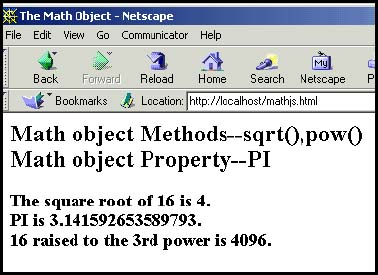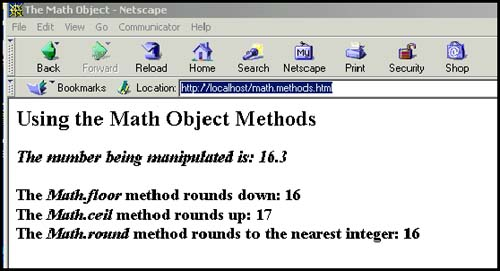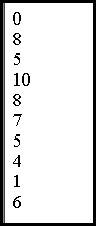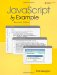# 9.5 The Math Object

### 9.5 The Math Object

The Math object allows you to work with more advanced arithmetic calculations, such as square root, trigonometric functions, logarithms, and random numbers , than are provided by the basic numeric operators. If you are doing simple calculations, you really won't need it.

Unlike other objects, you don't have to create an instance of the Math object with the new keyword. It is a built-in object and has a number of properties (see Table 9.5) and methods (see Table 9.6). The Math object always starts with an uppercase M.

##### Table 9.5. Math object properties.

Property

Value

Description

Math.E

2.718281828459045091

Euler's constant, the base of natural logarithms

Math.LN2

0.6931471805599452862

Natural log of 2

Math.LN10

2.302585092994045901

Natural log of 10

Math.LOG2E

1.442695040888963387

Log base-2 of E

Math.Log10E

0.4342944819032518167

Log base-10 of E

Math.PI

3.14592653589793116

Pi, ratio of the circumference of a circle to its diameter

Math.SQRT1_2

0.7071067811865475727

1 divided by the quare root of 2

Math.SQRT2

1.414213562373985145

Square root of 2

##### Table 9.6. Math object methods.

Method

Functionality

Math.abs(Number)

Returns the absolute (unsigned) value of Number

Math.acos(Number)

Arc cosine of Number , returns result in radians

Math.asin(Number)

Arc sine of Number , returns results in radians

Math.atan(Number)

Arctangent of Number , returns results in radians

Math.atan2(y,x)

Arctangent of y / x ; returns arctangent of the quotient of its arguments

Math.ceil(Number)

Rounds Number up to the next closest integer

Math.cos(Number)

Returns the cosign of Number in radians

Math.exp(x) [*]

Euler's constant to some power (see footnote)

Math.floor(Number)

Rounds Number down to the next closest integer

Math.log(Number)

Returns the natural logarithm of Number (base E)

Math.max(Number1, Number2)

Returns larger value of Number1 and Number2

Math.min(Number1, Number2)

Returns smaller value of Number1 and Number2

Math.pow(x, y)

Returns the value of x to the power of y ( x y ), where x is the base and y is the exponent

Math.random()

Generates pseudorandom number between 0.0 and 1.0

Math.round(Number)

Rounds Number to the closest integer

Math.sin(Number)

Arc sine of Number in radians

Math.sqrt(Number)

Square root of Number

Math.tan(Number)

Math.toString(Number)

Converts Number to string

[*] Returns the value of E x where E is Euler's constant and x is the argument passed to it. Euler's constant is approximately 2.7183.

#### Square Root, Power of, and Pi

The Math object comes with a number of common mathematical constants (all uppercase), such as PI and natural log values, as well as methods to find the square root of a number, the power of a number, and so on. Example 9.16 demonstrates how to use some of these properties; the output is shown in Figure 9.17.

##### Example 9.16
` <html>     <head><title>The Math Object</title></head>     <body>     <h2>Math object Methods--sqrt(),pow()<br>     Math object Property--PI</h2>     <P>     <script language="JavaScript"> 1  var num=16;  document.write("<h3>The square root of " +num+ " is "); 2       document.write(  Math.sqrt(num)  ,".<br>");         document.write("PI is "); 3       document.write(  Math.PI  );         document.write(".<br>"+num+" raised to the 3rd power is " ); 4       document.write(  Math.pow(num,3  ));         document.write(".</h3></font>");     </script>     </body></html> `
##### Figure 9.17. Output from Example 9.16.#### 9.5.1 Rounding Up and Rounding Down

There are three Math methods available for rounding numbers up or down. They are the ceil(), floor() , and round() methods (see Table 9.7 for examples). The differences between the methods might be confusing because all three methods truncate the numbers after the decimal point and return a whole number. If you recall, JavaScript also provides the parseInt() function, but this function truncates the number after the decimal point, without rounding either up or down.

##### The ceil() Method

The ceil() method rounds a number up to the next largest whole number and then removes any numbers after the decimal point; thus, 5.02 becomes 6 because 6 is the next largest number, and “5.02 becomes “5 because “5 is larger than “6.

##### The floor() Method

The floor() method rounds a number down to the next lowest whole number and then removes any numbers after the decimal point; thus, 5.02 now becomes 5, and “5.02 becomes “6.

##### The round() Method

The round() method rounds up only if the decimal part of the number is .5 or greater. Otherwise, it rounds down to the nearest integer; thus, 5.5 is rounded up to 6, and 5.4 is rounded down to 5.

Number

ceil()

floor()

round()

2.55

3

2

3

2.30

3

2

2

“2.5

“2

“3

“2

“2.3

“2

“3

“2

##### Example 9.17
` <html>     <head><title>The Math Object</title></head>     <body>     <h2>Rounding Numbers</h2>     <p>     <h3>     <script language="JavaScript"> 1  var num=16.3;  document.write("<I>The number being manipulated is: ", num,                    "</I><br><br>"); 2   document.write("The <I>Math.floor</I> method rounds down: " +  Math.floor(num)  + "<br>"); 3   document.write("The <I>Math.ceil</I> method rounds up: " +  Math.ceil(num)  +"<br>"); 4   document.write("The <I>Math.round</I> method rounds to\                    the nearest integer: " +  Math.round(num)  + "<br>");     </script>     </h3>     </body>     </html> `
##### Figure 9.18. Output from Example 9.17.#### 9.5.2 Generating Random Numbers

Random numbers are frequently used in JavaScript programs to produce random images (such as banners streaming across a screen), random messages, or random numbers (such as for lotteries or card games ). There are examples throughout this text where random numbers are used.

The Math object's random() method returns a random fractional number between 0 and 1 and is seeded with the computer's system time. (The seed is the starting number for the algorithm that produces the random number.) The Math object's floor() method truncates numbers after the decimal point and returns an integer.

##### Example 9.18
` <html><head><title>Random Numbers</title>     <font size="+1">     <script language="JavaScript"> 1       var n = 10; 2       for(i=0; i < 10;i++){         //  Generate random numbers between 0 and 10  3           document.write(  Math.floor(Math.random()* (n + 1)) +  "<br>");         }     </script>     </head>     <body></body>     </html> `

EXPLANATION

1. The variable n is assigned an initial value of 10 . This value will be the outside range of numbers randomly produced.

2. The for loop is entered and will cause the body of the block to be executed 10 times, thus producing 10 random numbers between 0 and 10.

3. The formula produces a random number between 0 and some number, n , using the Math object's random method. The output is shown in Figure 9.19.

##### Figure 9.19. Random numbers. Output from Example 9.18.JavaScript by Example (2nd Edition)
ISBN: 0137054890
EAN: 2147483647
Year: 2003
Pages: 150
Authors: Ellie Quigley

Similar book on Amazon